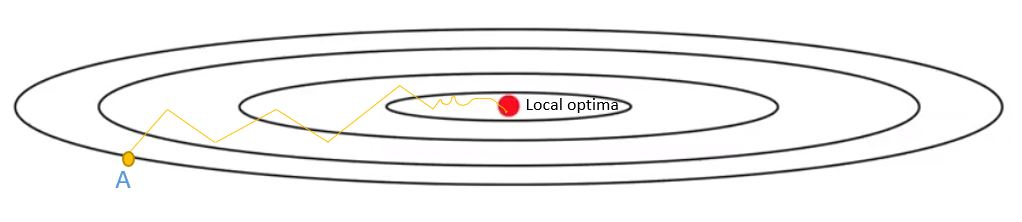/  Deep Learning Interview questions and answers   /  What do you mean by RMS Prop?## What do you mean by RMS Prop?

Ans: RMS Prop is an optimization technique which is not published yet used for Neural Networks. To know about RMS Prop, we need to know about R prop.

R Prop algorithm is used for full batch optimization. It tries to resolve the problem of the gradients with variable magnitudes. This makes difficult to find single global learning rate for algorithms. R Prop combines the idea of using sign of the gradient with adapting step size individually for each weight. But R prop does not really work when we have very large datasets and need to update mini batch weights.

It does not work because when we have small learning rate, it averages gradients over mini batches. With R Prop, weights increase more and decreases less. So, weight grows much larger.

To avoids this problem, we go for RMS Prop. The main idea of RMS Prop is to keep the moving average of squared gradients for each weight, and then we divide the gradient by square root of mean square.